Mathematics Commons™

17,867 Full-Text Articles 17,112 Authors 4,137,002 Downloads285 Institutions

All Articles in Mathematics

17,867 full-text articles. Page 462 of 528.

Some Topics In Roc Curves Analysis, 2011Georgia State University

Some Topics In Roc Curves Analysis, Xin Huang

Mathematics Dissertations

The receiver operating characteristic (ROC) curves is a popular tool for evaluating continuous diagnostic tests. The traditional definition of ROC curves incorporates implicitly the idea of "hard" thresholding, which also results in the empirical curves being step functions. The first topic is to introduce a novel definition of soft ROC curves, which incorporates the idea of "soft" thresholding. The softness of a soft ROC curve is controlled by a regularization parameter that can be selected suitably by a cross-validation procedure. A byproduct of the soft ROC curves is that the corresponding empirical curves are smooth.

The second topic is on ...

2011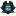East Tennessee State University

A Predictive Model Which Uses Descriptors Of Rna Secondary Structures Derived From Graph Theory., Alissa Ann Rockney

Electronic Theses and Dissertations

The secondary structures of ribonucleic acid (RNA) have been successfully modeled with graph-theoretic structures. Often, simple graphs are used to represent secondary RNA structures; however, in this research, a multigraph representation of RNA is used, in which vertices represent stems and edges represent the internal motifs. Any type of RNA secondary structure may be represented by a graph in this manner. We define novel graphical invariants to quantify the multigraphs and obtain characteristic descriptors of the secondary structures. These descriptors are used to train an artificial neural network (ANN) to recognize the characteristics of secondary RNA structure. Using the ANN ...

Arrangements Of Submanifolds And The Tangent Bundle Complement, 2011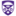The University of Western Ontario

Arrangements Of Submanifolds And The Tangent Bundle Complement, Priyavrat Deshpande

Electronic Thesis and Dissertation Repository

Drawing parallels with the theory of hyperplane arrangements, we develop the theory of arrangements of submanifolds. Given a smooth, finite dimensional, real manifold $X$ we consider a finite collection $\A$ of locally flat codimension $1$ submanifolds that intersect like hyperplanes. To such an arrangement we associate two posets: the \emph{poset of faces} (or strata) $\FA$ and the \emph{poset of intersections} $L(\A)$. We also associate two topological spaces to $\A$. First, the complement of the union of submanifolds in $X$ which we call the \emph{set of chambers} and denote by $\Ch$. Second, the complement of union of ...

Set: The Probabilities And Possibilities, 2011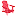University of Lynchburg

Set: The Probabilities And Possibilities, Tabitha K. Bollinger

Undergraduate Theses and Capstone Projects

The card game SET involves finding groups o f three cards called SETs. Choices are based upon the individual card characteristics, including shape, pattern, number, and color. Previously, the maximum number o f cards that can be played without creating a SET has been determined as 20 cards by extensive computer work. This report further explored the probabilities and possibilities o f the game. Using discrete mathematics and probability, we explored how many SETs are possible and what strategies led to the most points. Additionally, this project exercised undergraduate logic and reasoning to generalize the results in order to be ...

Complete Intersections In Toric Ideals, 2011Selected Works

Complete Intersections In Toric Ideals, Eduardo Cattani, Raymond Curran, Alicia Dickenstein

Eduardo Cattani

We present examples which show that in dimension higher than one or codimension higher than two, there exist toric ideals IA such that no binomial ideal contained in IA and of the same dimension is a complete intersection. This result has important implications in sparse elimination theory and in the study of the Horn system of partial differential equations.

2011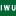Illinois Wesleyan University

Characterizations Of Orthogonal Generalized Gegenbauer-Humbert Polynomials And Orthogonal Sheffer-Type Polynomials, Tian-Xiao He

Scholarship

We present characterizations of the orthogonal generalized Gegen-bauer-Humbert polynomial sequences and the orthogonal Sheffer-type polynomial sequences. Using a new polynomial sequence transformation technique presented in , we give a method to evaluate the measures and their supports of some orthogonal generalized Gegenbauer-Humbert polynomial sequences.

2011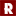Rose-Hulman Institute of Technology

Algebraic Solutions To Overdefined Systems With Applications To Cryptanalysis, Eric Crockett

Mathematical Sciences Technical Reports (MSTR)

Cryptographic algorithms are based on a wide variety of difficult problems in mathematics. One of these problems is finding a solution to a system of multivariate quadratic equations (MQ). A generalization of this problem is to find a solution to a system of higher order non-linear equations. Both of these problems are NP-hard over any field. Many cryptosystems such as AES, Serpent, Toyocrypt, and others can be reduced to some form of the MQ problem. In this paper we analyze the relinearization and XL algorithms for solving overdetermined systems of non-linear equations, as well as two variations of the XL ...

Rated Extremal Principles For Finite And Infinite Systems, 2011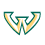Wayne State University

Rated Extremal Principles For Finite And Infinite Systems, Hung M. Phan, Boris S. Mordukhovich

Mathematics Research Reports

In this paper we introduce new notions of local extremality for finite and infinite systems of closed sets and establish the corresponding extremal principles for them called here rated extremal principles. These developments are in the core geometric theory of variational analysis. We present their applications to calculus and optimality conditions for problems with infinitely many constraints.

Hermite Polynomials And Sylvester Type Determinants, 2011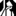University at Albany, State University of New York

Hermite Polynomials And Sylvester Type Determinants, Kelly C. Stange

Mathematics and Statistics

In this paper, we first recall Hermite polynomials, a particular family of orthogonal polynomials. We then evaluate their recurrence relations in terms of Sylvester type determinants of certain tridiagnoal matrix.

Strategic Treatment Interruptions During Imatinib Treatment Of Chronic Myelogenous Leukemia, 2011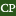California Polytechnic State University - San Luis Obispo

Strategic Treatment Interruptions During Imatinib Treatment Of Chronic Myelogenous Leukemia, Dana C. Paquin, Peter S. Kim, Peter P. Lee, Doron Levy

Mathematics

Although imatinib is an effective treatment for chronic myelogenous leukemia (CML), and nearly all patients treated with imatinib attain some form of remission, imatinib does not completely eliminate leukemia. Moreover, if the imatinib treatment is stopped, most patients eventually relapse (Cortes et al. in Clin. Cancer Res. 11:3425–3432, 2005). In Kim et al. (PLoS Comput. Biol. 4(6):e1000095, 2008), the authors presented a mathematical model for the dynamics of CML under imatinib treatment that incorporates the anti-leukemia immune response. We use the mathematical model in Kim et al. (PLoS Comput. Biol. 4(6):e1000095, 2008) to study ...

Bias In Monte Carlo Simulations Due To Pseudo-Random Number Generator Initial Seed Selection, 2011Beaumont Health System

Bias In Monte Carlo Simulations Due To Pseudo-Random Number Generator Initial Seed Selection, Jack C. Hill, Shlomo S. Sawilowsky

Theoretical and Behavioral Foundations of Education Faculty Publications

Pseudo-random number generators can bias Monte Carlo simulations of the standard normal probability distribution function with initial seeds selection. Five generator designs were initial-seeded with values from 10000HEX to 1FFFFHEX, estimates of the mean were calculated for each seed, the distribution of mean estimates was determined for each generator and simulation histories were graphed for selected seeds.

Third And Fourth Binomial Coefficients, 2011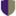Harvey Mudd College

Third And Fourth Binomial Coefficients, Arthur T. Benjamin, Jacob N. Scott '11

All HMC Faculty Publications and Research

While formulas for the sums of kth binomial coefficients can be shown inductively or algebraically, these proofs give little insight into the combinatorics involved. We prove formulas for the sums of 3rd and 4th binomial coefficients via purely combinatorial arguments.

2011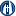George Fox University

Operator Splitting Method And Applications For Semilinear Parabolic Partial Differential Equations, R. Corban Harwood

Faculty Publications - Department of Mathematics and Applied Science

This dissertation presents a redefined operator splitting method used in solving semilinear parabolic partial differential equations. As one such form, the reaction-diffusion equation is highly prevalent in mathematical modeling. Besides being physically meaningful as a separation of two distinct physical processes in this equation, operator splitting simplifies the solution method in several ways. The super-linear speed-up of computations is a rewarding simplification as it presents great benefits for large-scale systems. In solving these semilinear equations, we will develop a condition for oscillation-free methods, a condition independent of the usual stability condition. This numerical consideration is important to fully embody our ...

2011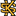Fitchburg State University

The Minimum-Norm Least-Squares Solution Of A Linear System And Symmetric Rank-One Updates, Xuzhou Chen, Jun Ji

Faculty Publications

In this paper, we study the Moore-Penrose inverse of a symmetric rank-one perturbed matrix from which a finite method is proposed for the minimum-norm least-squares solution to the system of linear equations Ax = b. This method is guaranteed to produce the required result.

2011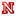University of Nebraska-Lincoln

On Morrey Spaces In The Calculus Of Variations, Kyle Fey

Dissertations, Theses, and Student Research Papers in Mathematics

We prove some global Morrey regularity results for almost minimizers of functionals of the form u → ∫Ω f(x, u, ∇u)dx. This regularity is valid up to the boundary, provided the boundary data are sufficiently regular. The main assumption on f is that for each x and u, the function f(x, u, ·) behaves asymptotically like the function h(|·|)α(x), where h is an N-function.

Following this, we provide a characterization of the class of Young measures that can be generated by a sequence of functions {fj} uniformly bounded in the Morrey space Lp, λ ...

2011The College at Brockport

Females In Mathematics: Creating An Environment That Aims To Lessen Anxiety And Promote Participation, Susan Rose Smalter

Education and Human Development Master's Theses

Self-worth theory of achievement motivation asserts that in a situation where poor performance is expected to show shortcomings in aptitude, students will withdraw in order to avoid the possibility of damaging their self-worth. It is in these settings where female students’ self-perception impacts their ability to participate and learn. Therefore, it is of utmost importance that we create classrooms, particularly within the mathematics and science arenas, where female students do not feel inferior to their male counterparts nor feel uncomfortable expressing their ideas – a place where they can respond and learn without consequence or damage to self-esteem. Quite simply, the ...

A Survey Of Modern Mathematical Cryptology, 2011The University of Tennessee

A Survey Of Modern Mathematical Cryptology, Kenneth Jacobs

Chancellor’s Honors Program Projects

No abstract provided.

Finding The Beat In Music: Using Adaptive Oscillators, 2011Harvey Mudd College

Finding The Beat In Music: Using Adaptive Oscillators, Kate M. Burgers

HMC Senior Theses

The task of finding the beat in music is simple for most people, but surprisingly difficult to replicate in a robot. Progress in this problem has been made using various preprocessing techniques (Hitz 2008; Tomic and Janata 2008). However, a real-time method is not yet available. Methods using a class of oscillators called relay relaxation oscillators are promising. In particular, systems of forced Hopf oscillators (Large 2000; Righetti et al. 2006) have been used with relative success. This work describes current methods of beat tracking and develops a new method that incorporates the best ideas from each existing method and ...

2011Harvey Mudd College

Markov Bases For Noncommutative Harmonic Analysis Of Partially Ranked Data, Ann Johnston

HMC Senior Theses

Given the result $v_0$ of a survey and a nested collection of summary statistics that could be used to describe that result, it is natural to ask which of these summary statistics best describe $v_0$. In 1998 Diaconis and Sturmfels presented an approach for determining the conditional significance of a higher order statistic, after sampling a space conditioned on the value of a lower order statistic. Their approach involves the computation of a Markov basis, followed by the use of a Markov process with stationary hypergeometric distribution to generate a sample.This technique for data analysis has become an accepted ...

Extending List Colorings Of Planar Graphs, 2011Harvey Mudd College

Extending List Colorings Of Planar Graphs, Sarah Loeb

HMC Senior Theses

In the study of list colorings of graphs, we assume each vertex of a graph has a specified list of colors from which it may be colored. For planar graphs, it is known that there is a coloring for any list assignment where each list contains five colors. If we have some vertices that are precolored, can we extend this to a coloring of the entire graph? We explore distance constraints when we allow the lists to contain an extra color. For lists of length five, we fix $W$ as a subset of $V(G)$ such that all vertices in ...